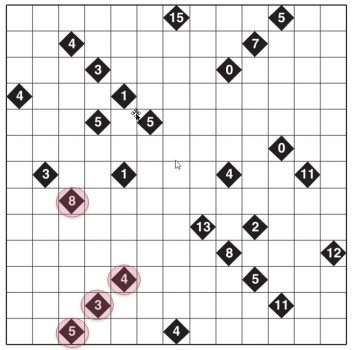# Follow the light – Clue 2

You must draw the following lines starting from each number:

RED 8 → Two squares to the left. Four squares to the right, and two squares to the top.

RED 4 → Four squares to the left.

RED 3 → Three squares to the left.

RED 5 → Two squares to the left. Three squares to the right.→